# Rotate a matrix by 90 degrees in an anticlockwise direction

Key takeaway: It is a famous matrix-based problem that can be solved in-place using the loop and properties of the matrix.

### Let’s understand the problem

Given an n x n square matrix, write a program to rotate it by 90 degrees in the anticlockwise direction. It is expected to rotate the matrix in place. Or in other words, we have to modify the input 2D matrix directly.

Examples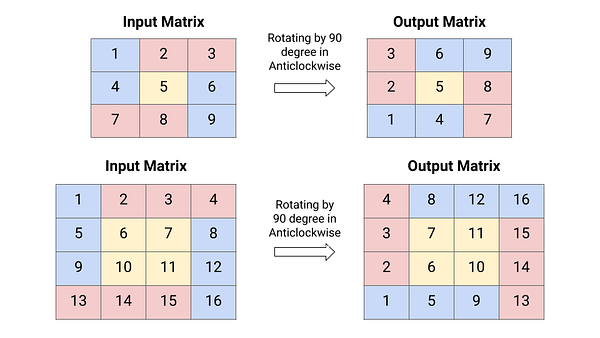Important note: Before moving to the solutions, we recommend learners solve this problem. If solved, then well done! We would like to hear your ideas in the comment. Otherwise, no problem, this is an opportunity to learn a new pattern in problem-solving!

### Discussed solution approaches

• A brute force approach  using extra space
• An efficient approach using the transpose of the matrix
• An efficient approach  using nested loops (In-place)

### A brute force approach  using extra space

Solution Idea and Steps

If we observe input and output matrices, then the following pattern would be visible: The first row has turned into the first column in reverse order. Similarly, second, third, …rows have turned into their respective column in reverse order. For example:

• The first row in the input matrix: 1, 2, 3, 4,
• The first column in the output matrix: 4, 3, 2, 1

So one basic idea would be simple: use an extra matrix of the same size and directly copy elements from the original matrix according to the above observation.

• The first row of the input matrix = The first column of the output matrix in the reverse order
• The second row of the input matrix = The second column of the output matrix in the reverse order and so .….. on.
• The last row of the input matrix = The last column of the output matrix in the reverse order.

Solution Pseudocode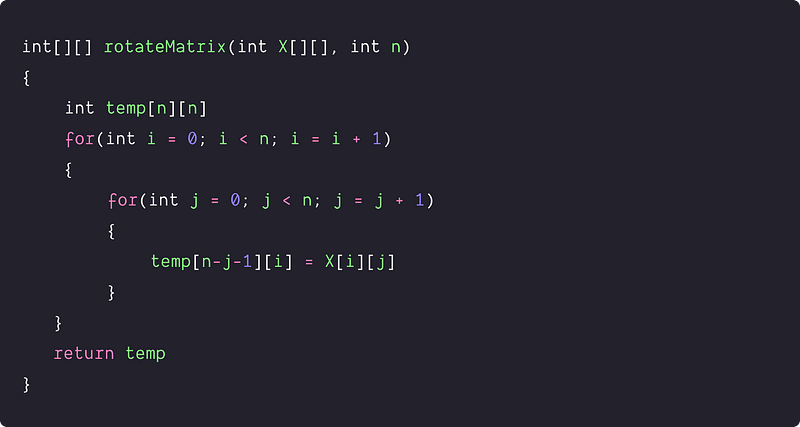Time and space complexity analysis

The above algorithm uses traversal of the matrix using two nested loops which takes O(n²) time, and the use of extra matrix takes O(n²) space. Hence, Time complexity = O(n²) and Space complexity = O(n²).

As given in the problem description, can we solve the problem without using extra space or in-place? Can we observe some pattern in the anticlockwise rotation of the matrix? Let's think!

### An efficient approach  using the transpose of the matrix

Solution Idea

If we deep dive into the input and output matrix, we can find patterns to rotate the matrix by swapping the values only. Let’s go through the same observation from the above approach. After the rotation of a matrix by 90 degrees anticlockwise:

• The first row of the input matrix = The first column of the output matrix in the reverse order
• The last row of the input matrix = The last column of the output matrix in the reverse order

So if we take the transpose of the input matrix, then:

• The first row of the input matrix = The first column of the output matrix.
• The last row of the matrix = The last column of the output matrix.

If we compare the output matrix with the transpose of the input matrix, then the solution idea would be: if we take transpose the input matrix and reverse each column, we will get the desired matrix rotated by 90 degrees in an anticlockwise direction (Think!)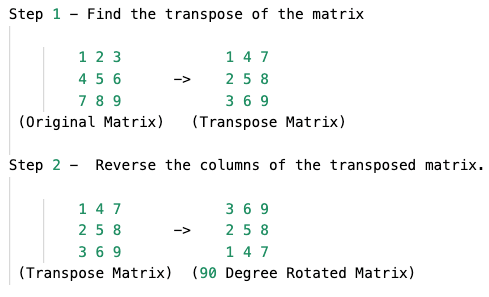Solution Steps

• Convert the given matrix into its transpose :  A transpose of a matrix is when the matrix is flipped over its diagonal, i.e., the row index of an element becomes the column index and vice versa. So, we need to swap elements at position X[i][j] with the element at X[j][i].

``````for (int i = 0; i < n; i = i + 1)
{
for (int j = i; j < n; j = j + 1)
{
swap(X[i][j], X[j][i])
}
}
``````
• Then, reverse the transposed matrix column-wise —we run two nested loops where the outer loop scans each column from 0 to n-1 and the inner loop reverses each column by swapping the values.

``````int i = 0, j = 0, column = 0
while(column < n)
{
i = 0, j = n-1
while(i < j)
{
swap(X[i][column], X[j][column])
i = i + 1
j = j - 1
}
column = column + 1
}
``````

Solution Pseudocode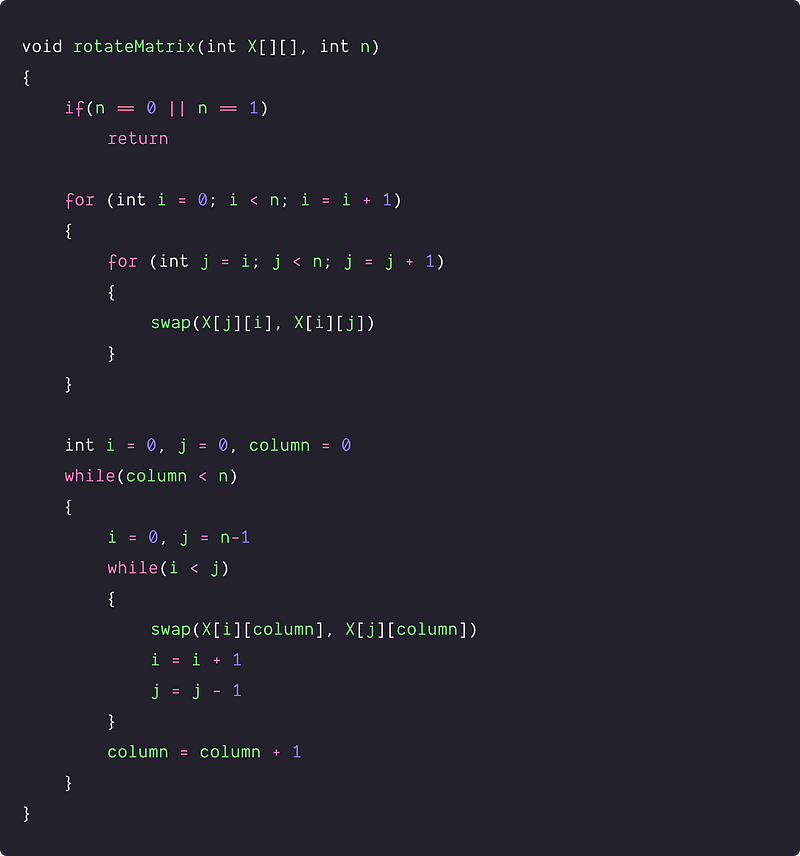Time and space complexity analysis

We are traversing the input matrix twice using nested loops. Time complexity = Time complexity of converting input matrix into a transpose + Time complexity of reversing transposed matrix column-wise = O(n²) + O(n²) = O(n²). Space Complexity = O(1), as no extra memory is needed.

### An efficient approach  using nested loops (In-place)

Solution Idea and Steps

There is another idea that can solve this problem in place. If we track the initial and final position of each element after the rotation then we can get a solution pattern.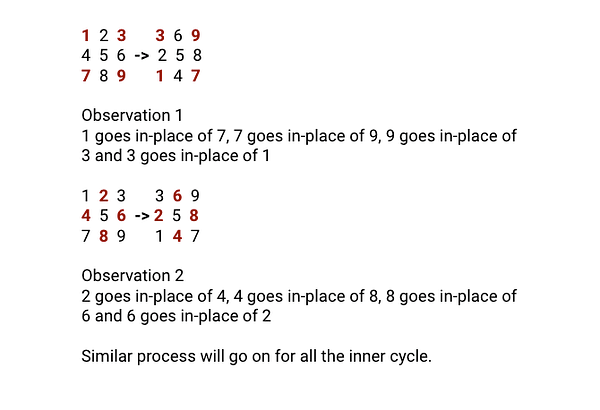So here are the critical conservations for the solution:

1. There is a total of n/2 squares in an n*n matrix and we can process each square one at a time using a nested loop. We first process the first square formed by its first row, last column, last row, and first column. Then we process the second square formed by the second row, second-last column, second-last row, and second column. And so on, we move from the outer square to the inner square.
2. In each square, elements are moving in a cycle of 4 elements. We swap the elements involved in an anticlockwise direction for each cycle, i.e., from right to the top, top to left, left to bottom, bottom to the right. In general, the position of elements in the any cycle is : (n-1-j, i), (i, j), (j, n-1-i) and (n-1-i, n-1-j). So we rotate these four elements by 90 degrees in anticlockwise order:
3. Element at position (n-1-j, i) will go to position (i, j).
4. Element at position (i, j) will go to position (j, n-1-i).
5. Element at position (j, n-1-i) will go to position (n-1-i, n-1-j).
6. Element at position (n-1-i, n-1-j) will go to position (n-1-j, i).

Solution Pseudocode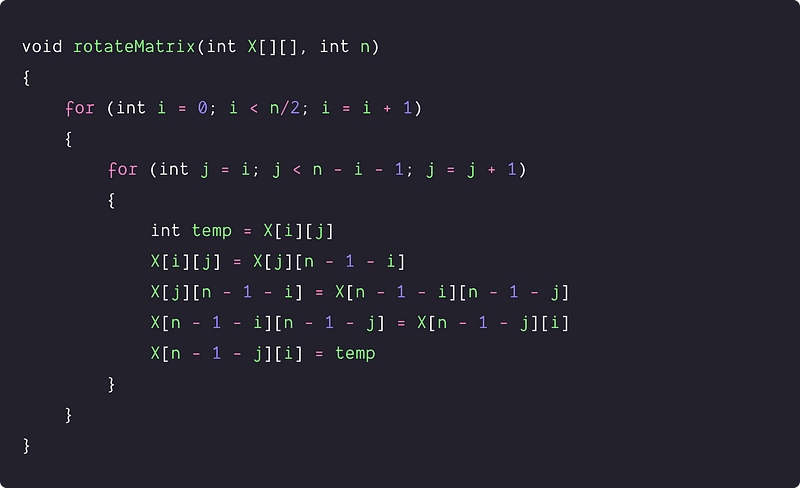Time and space complexity analysis

We are traversing each element of the matrix only once using a nested loop and fixing its correct position. Time Complexity = O(n²). Space Complexity = O(1), as no extra memory is needed.

### Critical ideas to think!

• Can we solve the problem by first reversing each row and then doing transpose of the matrix? If yes, then write code for it.
• How can we rotate the matrix in a clockwise direction?
• Can we find transpose in-place using some different approach?
• How do we generalize the solution for a m x n rectangular matrix?
• Can we design a general algorithm for rotating matrix by multiple of 90 degrees?

### Comparisons of time and space complexities

• Extra Space: Time = O(n^2), Space = O(n^2)
• Matrix Transpose: Time = O(n^2), Space = O(1)
• Nested loops (In-place): Time = O(n^2), Space = O(1)

### Matrix-based problems for practice

Thanks to Navtosh Kumar for his contribution in creating the first version of the content. Please write comments if you find an error or you want to share more insights about the topic. Enjoy learning, Enjoy coding, Enjoy algorithms!

Get well-designed application and interview centirc content on ds-algorithms, machine learning, system design and oops. Content will be delivered weekly.